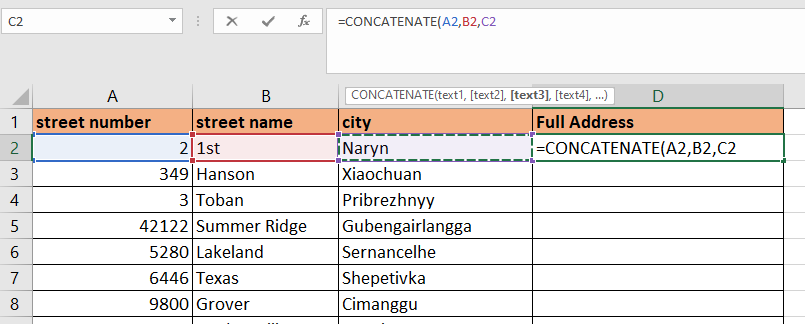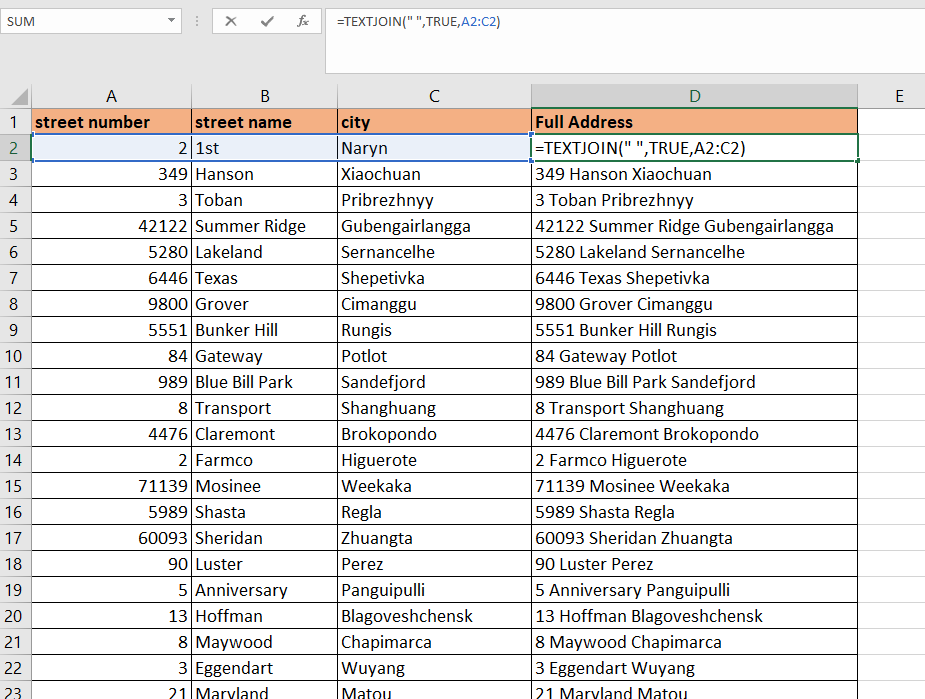# How to Stack Multiple Columns into One Column in Excel

The CONCAT and TEXTJOIN functions are useful when you need to combine multiple columns of text values into a single column in Excel.

When working with data, users may want to combine strings from different columns into a single column. Excel offers multiple ways to perform this operation on your spreadsheet.

Let’s take a look at a quick example of a dataset where we can use the Excel functions to combine columns.

In the example below, we have address data separated by street number, street name, and city. Given that we want to copy the complete address for each entry easily, we’ve added another column called Full Address. How can we generate the values for this column?With the `CONCAT` function, we can select multiple cells as arguments and return a single string that concatenates each cell’s value. We can also use the more advanced `TEXTJOIN` function. This function asks us to provide a delimiter to separate each string.

These methods will be useful for many data types, including name data. We can also use these methods to generate our own comma-separated lists.

Now that we know which functions we can use to perform this operation, let’s look at a sample spreadsheet that uses these functions to combine columns.

## A Real Example of Stacking Multiple Columns into One Column in Excel

Let’s take a look at a real example that uses the `CONCAT` function in an Excel spreadsheet to combine three different columns into one.

The example below shows how we can use the `CONCAT` function to return a string that combines text values from different strings. The function can accept both text values and cell references as arguments.To get the values in Column D, we just need to use the following formula:

`=CONCAT(A2,” “,B2,” “,C2)`

We can also write the formula above using the ampersand symbol:

`=A2&” “&B2&” “&C2`

Since we use the space character consistently between each string, we can also use the `TEXTJOIN` function.

`=TEXTJOIN(“ “, TRUE, A2:C2)`

The first argument of `TEXTJOIN` refers to the delimiter to use when joining strings. The second argument indicates whether we should ignore empty cells. The third argument refers to the cells we want to combine into a single string.

In the second example below, we used the `TEXTJOIN` function to create a comma-separated list from three columns.We can create the comma-separated list seen above using the following formula:

`=TEXTJOIN(", ";TRUE;F2:H2)`

If you’re ready to try out these text functions in Excel to combine columns, head over to the next section to learn more.

## How to Combine Multiple Columns into One Column in Excel

This section will guide you through each step needed to combine multiple columns into a single column. You’ll learn how we can use `CONCATENATE` and `TEXTJOIN` functions to combine multiple strings with arbitrary delimiters.

Follow these steps to stack multiple columns into one using the `CONCATENATE` function:

1. First, type in the `CONCATENATE` function into the target cell. Each argument in this function must be a string or cell reference.2. Hit the Enter key to return the concatenated string. The function combines the strings in the order they were added as arguments.3. We can also add a space between text by providing the necessary string in our arguments.4. We can also use the `TEXTJOIN` function to concatenate values with a provided delimiter. In the example below, we have added a space in between the values in the range A2:C2.1. Can you format strings before combining them?
You can use the `TEXT` function if you want to format each cell value a certain way before combining. For example, `TEXT(0.649, “0.0%”)` will show the decimal as ‘28.5%’ in the final string.
2. Why does my formula return a value error?
If your formula returns a #VALUE! error, you may have exceeded the maximum number of characters for a single cell. The cell limit in Excel is 32,767 characters.
3. What is the difference between the CONCAT and CONCATENATE function?
`CONCAT` is a newer function compared to the `CONCATENATE` function. The `CONCAT` function addresses that the `CONCATENATE` function cannot select a range of strings as an argument.
Although both of these functions are still usable due to backward compatibility, it is still best to use `CONCAT` as it is the newer function.

That’s all you need to remember to stack multiple columns into a single column in Excel. This step-by-step guide shows how you can use the `CONCAT` and `TEXTJOIN` functions to join multiple fields together into a single string.

These functions are one of many built-in functions in Excel that can manipulate text. With so many other Excel functions out there, you can surely find one that suits your use case.

Are you interested in learning more about what Excel can do? Make sure to subscribe to our newsletter to be the first to know about the latest guides and tutorials from us.### Get emails from us about Excel.

Our goal this year is to create lots of rich, bite-sized tutorials for Excel users like you. If you liked this one, you'd love what we are working on! Readers receive ✨ early access ✨ to new content.

##### You May Also Like## How to Perform Matrix Multiplication in Excel

This guide will explain how to perform matrix multiplication in Excel using the MMULT function. The rules for…## How to Do Conditional Formatting if Between Two Values in Excel

This guide will explain how to do conditional formatting if between two values in Excel. Since it has…## How to Calculate Root Mean Square Error in Excel

This guide will discuss how to calculate the root mean square error in Excel using three simple and…## How to Delete Filtered Rows in Excel

This guide will explain how to delete filtered rows in Excel. Since it has several built-in functions and…